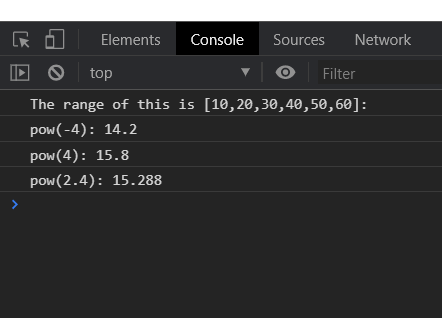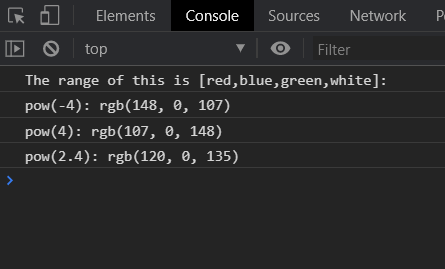# D3.js pow.range() Function

• Last Updated : 23 Aug, 2020

The pow.range() function in d3.js is used to set the scale’s range to the specified array of values that must contain two or more than two values. The elements in the range can be number or string.

Syntax:

Hey geek! The constant emerging technologies in the world of web development always keeps the excitement for this subject through the roof. But before you tackle the big projects, we suggest you start by learning the basics. Kickstart your web development journey by learning JS concepts with our JavaScript Course. Now at it's lowest price ever!

```pow.range([range]);
```

Parameters: This function takes a single parameter that is given above and described below.

• [range]: This is an array that contains the range for the domain specified.

Return Values: This function does not return any value.

Example 1:

## html

 ```<``html` `lang``=``"en"``>`` ` `<``head``>``    ``<``meta` `charset``=``"UTF-8"` `/>``    ``<``meta` `name``=``"viewport"` `path1tent``=``        ``"width=device-width,initial-scale=1.0"` `/>`` ` `    ``<``script` `src``=``"https://d3js.org/d3.v4.min.js"``>``    `````` ` `<``body``>``    ``<``script``>``        ``var pow = d3.scalePow()`` ` `            ``// Setting domain for the scale.``            ``.domain([-10, 10])``         ` `            ``// Range of numbers``            ``.range([10, 20, 30, 40, 50, 60])``            ``.exponent(2);``        ``console.log("The range of this is "``                ``+ "[10,20,30,40,50,60]: ");``        ``console.log("pow(-4): " + pow(-4));``        ``console.log("pow(4): " + pow(4));``        ``console.log("pow(2.4): " + pow(2.4));``    `````` ` ``

Output:Example 2:

## html

 ```<``html` `lang``=``"en"``>`` ` `<``head``>``    ``<``meta` `charset``=``"UTF-8"` `/>``    ``<``meta` `name``=``"viewport"` `path1tent``=``        ``"width=device-width,initial-scale=1.0"` `/>`` ` `    ``<``script` `src``=``"https://d3js.org/d3.v4.min.js"``>``    `````` ` `<``body``>``    ``<``script``>``        ``var pow = d3.scalePow()`` ` `            ``// Setting domain for the scale.``            ``.domain([-10, 10])``         ` `            ``// Range of colors``            ``.range(["red", "blue", "green", "white"])``            ``.exponent(2);``        ``console.log("The range of this is"``            ``+ " [red,blue,green,white]: ");``        ``console.log("pow(-4): " + pow(-4));``        ``console.log("pow(4): " + pow(4));``        ``console.log("pow(2.4): " + pow(2.4));``    `````` ` ``

Output:My Personal Notes arrow_drop_up